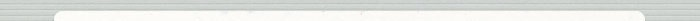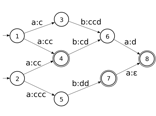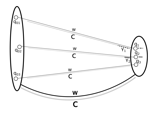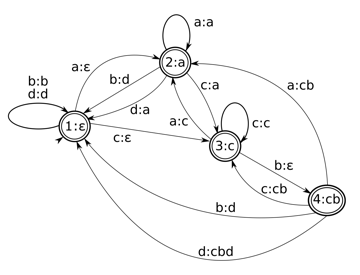Finite-State Techniques

Automata, Transducers and BimachinesCambridge University Press. ISBN: 9781108485418Finite-state techniques provide theoretically elegant and computationally efficient solutions for various (hard, non-trivial) problems in text and natural language processing. Due to its importance in many fundamental applications, the theory of finite-state automata and related finite-state machines has been extensively studied and its development still continues.

This textbook describes the basics of finite state technology, following a combined mathematical and implementational point of view. It is written for advanced undergraduate and graduate students in computer science, computational linguistics and mathematics. Though concepts are introduced in a mathematically rigorous way and correctness proofs for all procedures are given, the book is not meant as a purely theoretical introduction to the subject. The ultimate goal is to bring students to a position where they can both understand and implement complex finite-state based procedures for practically relevant tasks.